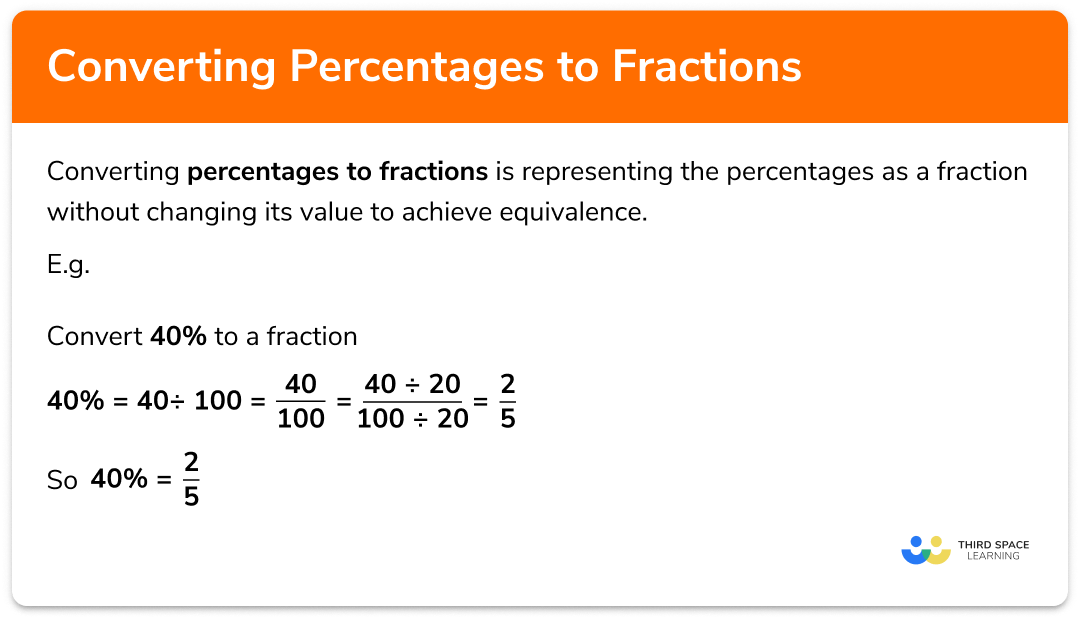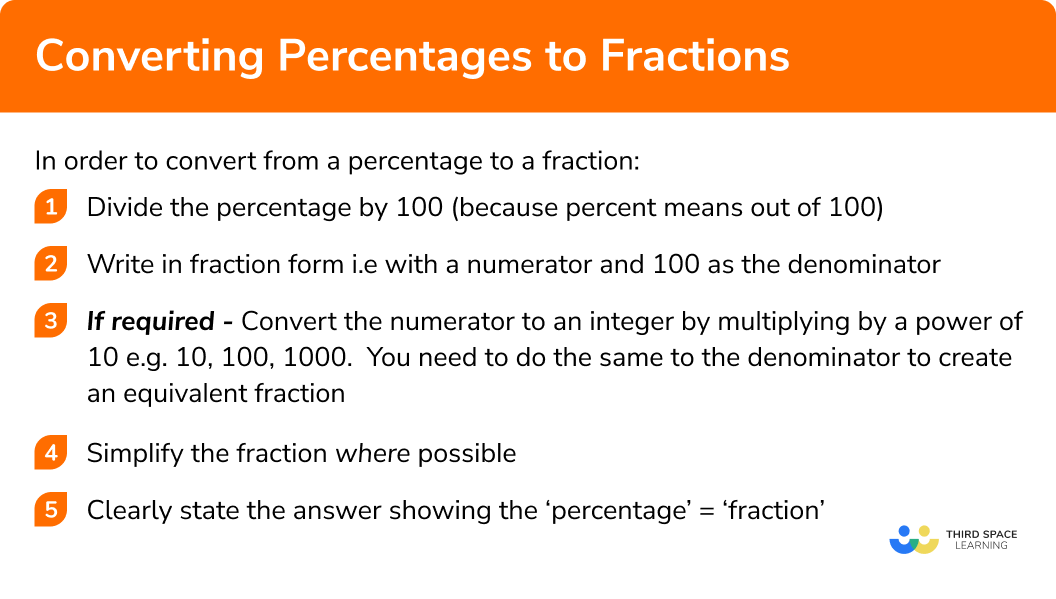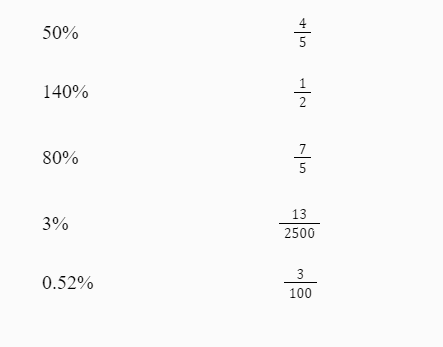GCSE Maths Number FDP Converting FDP

Percentages To Fractions

# Percentages To Fractions

Here we will learn about converting percentages to fractions.

There are also percentages to fractions worksheets based on Edexcel, AQA and OCR exam questions, along with further guidance on where to go next if you’re still stuck.

## What is converting percentages to fractions?

Converting percentages to fractions is representing the percentages as a fraction without changing its value to achieve equivalence.

E.g.

$25\% =\frac{1}{4} \\ 45\% =\frac{9}{20} \\ 33.\dot{3}\% =\frac{1}{3} \\ 80\% =\frac{4}{5}$

### What is converting percentages to fractions?## How to convert percentages to fractions

In order to convert a percentage to a fraction:

1. Divide the percentage by a hundred (because percent means out of \pmb{100}).
2. Write in fraction form i.e with a numerator and \pmb{100} as the denominator.
3. If required, convert the numerator to an integer by multiplying by a power of \pmb{10}
E.g. \pmb{10}, \pmb{100}, \pmb{1000}. You need to do the same to the denominator to create an equivalent fraction.
4. Simplify the fraction (where possible).
5. Clearly state the answer showing the ‘percentage’ = ‘fraction’.

### Explain how to convert from a percentage to a fraction in 5 steps## Converting percentages to fractions examples

### Example 1: converting a simple percentage to a fraction (without simplifying)

Convert 7% to a fraction.

1. Divide the percentage by a hundred.

$7\div100$

2Write in fraction form.

$7\div100=\frac{7}{100}$

3If required, convert the numerator to an integer by multiplying by a multiple of \pmb{10} . You need to do the same to the denominator to create an equivalent fraction.

The numerator is already an integer so you do not need to multiply the numerator and denominator by a multiple of 10.

4Simplify the fraction.

This fraction cannot be simplified because the only factor 7 and 100 share is 1.

5Clearly state the answer showing ‘percentage’ = ‘fraction’.

$7\%=\frac{7}{100}$

We can read this as ‘7 hundredths’ or ‘7 out of 100’.

This is a decimal fraction as the denominator is a power of 10.

### Example 2: converting a percentage to a fraction (simplifying)

Convert 40% to a fraction, give your answer in its simplest form.

$40\div100$
$40\div100=\frac{40}{100}$

The numerator is already an integer so you do not need to multiply the numerator and denominator by a multiple of 10.

$\frac{40}{100}$

can be simplified by dividing the numerator and denominator by 20 (the highest common factor of 20 and 100):

$\frac{40\div20}{100\div20}=\frac{2}{5}$
$40\%=\frac{2}{5}$

### Example 3: converting a percentage to a fraction

Convert 60.2% to a fraction.

$60.2\div100$
$60.2\div100=\frac{60.2}{100}$

The lowest value in the number 60.2 is the 2 ‘tenths’ in the first decimal place. This means if we multiply 60.2 by 10 we will get the integer value 602.
We need to multiply the numerator and the denominator by the same value.

$\frac{60.2}{100}=\frac{60.2\times10}{100\times10}=\frac{602}{1000}$

$\frac{62}{1000}$

can be simplified by dividing the numerator and denominator by 2 (the highest common factor of 602 and 1000):

$\frac{602\div2}{1000\div2}=\frac{301}{500}$
$60.2\%=\frac{301}{500}$

### Example 4: converting a percentage to a fraction

Convert 120% to a fraction. Give you answer as a improper fraction.

$120\div100$
$120\div100=\frac{120}{100}$

The numerator is already an integer so you do not need to multiply the numerator and denominator by a multiple of 10.

$\frac{120}{100}$

can be simplified by dividing the numerator and denominator by 20 (the highest common factor of 120 and 100):

$\frac{120\div20}{100\div20}=\frac{6}{5}$
$120\%=\frac{6}{5}$

This is an improper fraction as the numerator is greater than the denominator.

### Example 5: converting a percentage to a fraction

Convert 150% to a fraction. Give you answer as mixed number.

$150\div100$
$150\div100=\frac{150}{100}$

The numerator is already an integer so you do not need to multiply the numerator and denominator by a multiple of 10.

$\frac{150}{100}$

can be simplified by dividing the numerator and denominator by 50 (the highest common factor of 150 and 100):

$\frac{150\div50}{100\div50}=\frac{3}{2}$
$150\%=\frac{3}{2}$
$\frac{3}{2}$

This is an improper fraction, we need to give the answer as a mixed number.

$\frac{3}{2}=1\frac{1}{2}$

Therefore,

$150\%=1\frac{1}{2}$

Step by step guide: Improper Fractions and Mixed Numbers

### Example 6: converting a percentage to a fraction

Convert 0.008% to a fraction, give your answer in its simplest form.

$0.008\div100$
$0.008\div100=\frac{0.008}{100}$

The lowest value in the number 0.008 is the third digit after the decimal point, 8 ‘thousandths’. This means if we multiply 0.008 by 1000 we will get the integer value 8.
We need to multiply the numerator and the denominator by the same value.

$\frac{0.008\times1000}{100\times1000}=\frac{8}{100000}$
$\frac{8}{100000}$

can be simplified by dividing the numerator and denominator by 8 (the highest common factor of 8 and 100000):

$\frac{8\div8}{100000\div8}=\frac{1}{12500}$
$0.008\%=\frac{1}{12500}$

### Common misconceptions

• Multiplying by an incorrect multiple of \pmb{10}

You must multiply by a power 10 (e.g 10, 100 or 1000) that results in the numerator being an integer (a whole number). Use your knowledge of place value to help decide which multiple of 10 to multiple by.

E.g.

0.003 × 10 = 0.03. This is not an integer.

0.003 × 1000 = 3. This is an integer.

• Simplifying the fraction

Often questions will say “give your answer in the simplest form” so we need to check whether the fraction can be simplified. To simplify a fraction we need to divide the numerator and the denominator by a common factor.

• Not multiplying the denominator by the same number as the numerator

When you multiply the numerator by a multiple of 10 you must do the same for the denominator, otherwise you are changing the value of the fraction.

• Converting between a mixed number and an improper fraction

A common error is to incorrectly convert between numbers in different forms
E.g. mixed numbers and improper fractions.

Percentages to fractions is part of our series of lessons to support revision on comparing fractions, decimals and percentages. You may find it helpful to start with the main comparing fractions, decimals and percentages lesson for a summary of what to expect, or use the step by step guides below for further detail on individual topics. Other lessons in this series include:

### Practice percentages to fractions questions

1. Convert 10 % to a fraction in its simplest form

\frac{10}{100}\frac{1}{10}\frac{0.1}{1}\frac{0.01}{100}10 % can be written as \frac{10}{100} . We can simplify this by dividing both the numerator and denominator by 10 .

2. Convert 20 % to a fraction in its simplest form

\frac{20}{100}\frac{2}{10}\frac{1}{5}\frac{0.4}{1}20% can be written as \frac{20}{100} . We can simplify this by dividing both the numerator and denominator by 5 .

3. Convert 130% to a fraction in its simplest form

\frac{1.3}{1}\frac{13}{10}\frac{130}{100}\frac{3}{10}130 % can be written as \frac{130}{100} . We can simplify this by dividing both the numerator and denominator by 10 .

4. Convert 0.6 % to a fraction in its simplest form

\frac{0.006}{1}\frac{6}{1}\frac{6}{1000}\frac{3}{500}0.6% can be written as \frac{0.6}{100} . We then need to multiply both the numerator and denominator by 10 to make the numerator an integer. We can simplify this by dividing both the numerator and denominator by 2 .

5. Convert 3005 % to a fraction in its simplest form

\frac{601}{20}\frac{1}{20}3005\frac{3005}{1000}3005 % can be written as \frac{3005}{100} . We can simplify this by dividing both the numerator and denominator by 5 .

6. Which of the below is the fractional equivalent of 12 %

\frac{3}{25}\frac{12}{100}\frac{6}{50}\frac{12}{10}12 % can be written as \frac{12}{100} . We can simplify this by dividing both the numerator and denominator by 4 .

### Percentages to fractions GCSE questions

1. Convert each of the following percentages to fractions:

a) 90%
b) 25%
c) 9%
d) 0.9%
e) 990%

All answers must be given in their simplest form.

(5 marks)

a) \frac{9}{10}

(1)

b) \frac{1}{4}

(1)

c) \frac{9}{100}

(1)

d) \frac{9}{1000}

(1)

e) \frac{99}{10}

(1)

2. Convert each of the following percentages to fractions:

a) 54%
b) 114%
c) 18.2%

All answers must be given in their simplest form.

(6 marks)

a) \frac{54}{100}

(1)

\frac{27}{50}

(1)

b) \frac{114}{100}

(1)

\frac{57}{50}

(1)

c) \frac{182}{100}

(1)

\frac{91}{500}

(1)

3. Match the percentages to the fractions:a) 50\%=\frac{1}{2}

(1)

b) 140\%=\frac{7}{5}

(1)

c) 80\%=\frac{4}{5}

(1)

d) 3\%=\frac{3}{100}

(1)

e) 0.52\%=\frac{13}{2500}

(1)

4. Write 88.8 % as a fraction in its simplest form.

(2 marks)

\frac{88.8}{100}

(1)

\frac{111}{125}

(1)

## Learning checklist

You have now learned how to:

• Convert a percentage to a fraction

## Still stuck?

Prepare your KS4 students for maths GCSEs success with Third Space Learning. Weekly online one to one GCSE maths revision lessons delivered by expert maths tutors.

Find out more about our GCSE maths tuition programme.